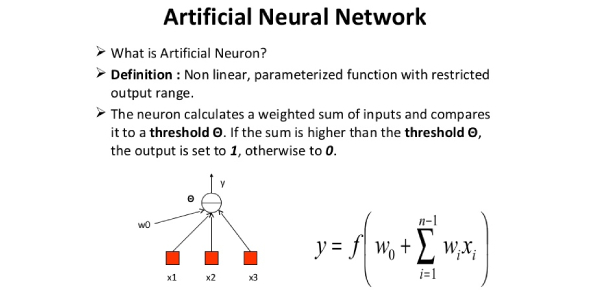# Applied Soft Computing - Post Assessment

10 Questions | Attempts: 3535SettingsIf you are a software developer, one of the things that you need to be conversant with is the topic of soft computing and artificial neural networks. The introduction of these techniques has helped greatly in easing work and diagnosis of errors in a system. Think you understand the topic? Do take up the quiz below and get to find out!

• 1.
An associative network is
• A.

A neural network that contains no loop

• B.

A neural network that contains feedback

• C.

A neural network that has only one loop

• D.

None of These

• 2.
What are the 2 types of learning
• A.

Improvised and unimprovised

• B.

Supervised and unsupervised

• C.

Layered and unlayered

• D.

None of the above

• 3.
Neural Computing ____________
• A.

Mimics human brain

• B.

• C.

Both (1) and (2)

• D.

None of the above

• 4.
Fuzzy Computing ______________
• A.

Mimics human behaviour

• B.

Does not deal with 2 valued logic

• C.

Deals with information which is vague, imprecise, uncertain, ambiguous, inexact, or probabilistic

• D.

All of the above

• 5.
Genetic Algorithm is a part of___________
• A.

Evolutionary Computing

• B.

Inspired by Darwin's theory about evolution - "survival of the fittest"

• C.

Are adaptive heuristic search algorithm based on the evolutionary ideas of natural selection and genetics

• D.

All of the above

• 6.
Genetic algorithms are heuristic methods that do not guarantee an optimal solution to a problem
• A.

True

• B.

False

• 7.
Conventional Artificial Intelligence is different from soft computing in the sense________
• A.

Conventional Artificial Intelligence deal with prdicate logic where as soft computing deal with fuzzy logic

• B.

Classical AI methods are limited by symbols where as soft computing is based on empirical data

• C.

Both (a) and (b)

• D.

None of the above

• 8.
Artificial neural network used for___________
• A.

Pattern Recognition

• B.

Classification

• C.

Clustering

• D.

All of these

• 9.
Japanese were the first to utilize fuzzy logic practically on high-speed trains in Sendai.
• A.

True

• B.

False

• 10.
Fuzzy logic is usually represented as
• A.

IF-THEN-ELSE rules

• B.

IF-THEN rules

• C.

Both IF-THEN-ELSE rules & IF-THEN rules

• D.

None of the mentioned

## Related TopicsBack to top
×

Wait!
Here's an interesting quiz for you.